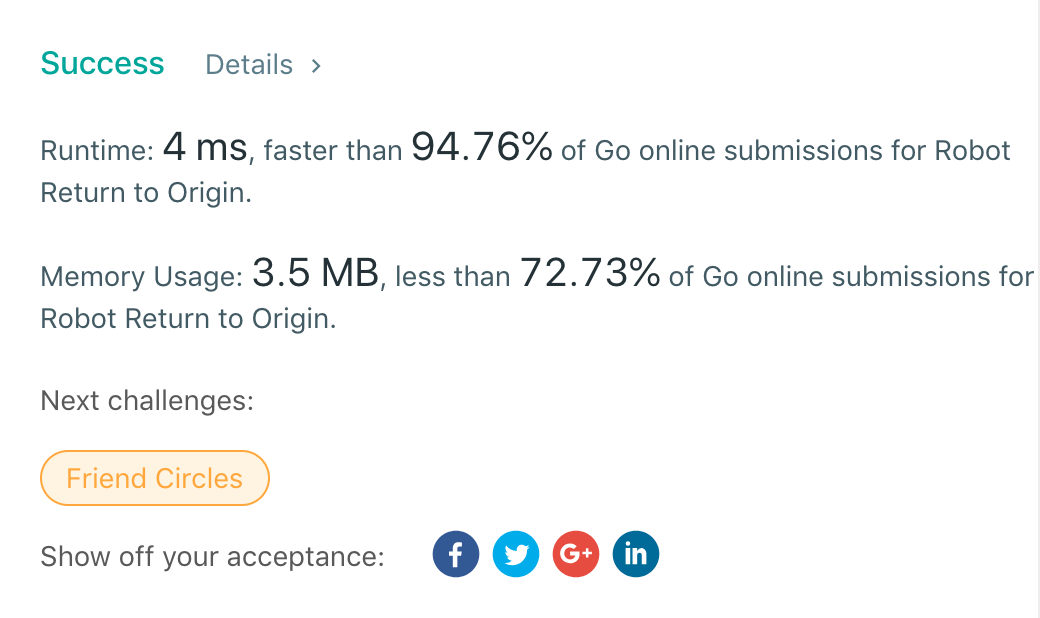# LeetCode算法题657

## 原题

There is a robot starting at position (0, 0), the origin, on a 2D plane. Given a sequence of its moves, judge if this robot ends up at (0, 0) after it completes its moves.

The move sequence is represented by a string, and the character moves[i] represents its ith move. Valid moves are R (right), L (left), U (up), and D (down). If the robot returns to the origin after it finishes all of its moves, return true. Otherwise, return false.

Note: The way that the robot is “facing” is irrelevant. “R” will always make the robot move to the right once, “L” will always make it move left, etc. Also, assume that the magnitude of the robot’s movement is the same for each move.

### Example 1:

Input: “UD”
Output: true
Explanation: The robot moves up once, and then down once. All moves have the same magnitude, so it ended up at the origin where it started. Therefore, we return true.

### Example 2:

Input: “LL”
Output: false
Explanation: The robot moves left twice. It ends up two “moves” to the left of the origin. We return false because it is not at the origin at the end of its moves.

## 实现

### Golang实现

https://github.com/cook-coder/my-leetcode-solution/tree/master/easy/657

## 成绩